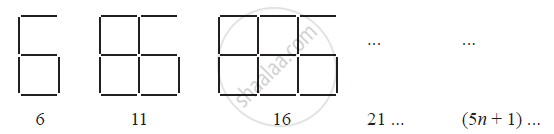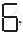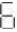Share

# Observe the Patterns of Digits Made from Line Segments of Equal Length. You Will Find Such Segmented Digits on the Display of Electronic Watches Or Calculators. - CBSE Class 7 - Mathematics

ConceptUsing Algebraic Expressions – Formulas and Rules

#### Question

Observe the patterns of digits made from line segments of equal length. You will find such segmented digits on the display of electronic watches or calculators.If the number of digits formed is taken to be n, the number of segments required to
form n digits is given by the algebraic expression appearing on the right of each pattern.

How many segments are required to form 5, 10, 100 digits of the kind −#### Solution

It is given that the number of segments required to form n digits of the kindis (5n + 1)

Number of segments required to form 5 digits = (5 × 5 + 1)

= 25 + 1 = 26

Number of segments required to form 10 digits = (5 × 10 + 1)

= 50 + 1 = 51

Number of segments required to form 100 digits = (5 × 100 + 1)

= 500 + 1 = 501

Is there an error in this question or solution?

#### APPEARS IN

NCERT Solution for Mathematics for Class 7 (2018 to Current)
Chapter 12: Algebraic Expressions
Ex. 12.40 | Q: 1.1 | Page no. 246
Solution Observe the Patterns of Digits Made from Line Segments of Equal Length. You Will Find Such Segmented Digits on the Display of Electronic Watches Or Calculators. Concept: Using Algebraic Expressions – Formulas and Rules.
S